# Examples on Permutations and Combinations Set 3

Go back to  'P & C, Binomial Theorem'

Example – 13

12 person are sitting in a row. In how many ways can we select 4 persons out of this group such that no two are sitting adjacent in the row?

Solution:   To make the question more clear, here’s a valid and a non-valid selection: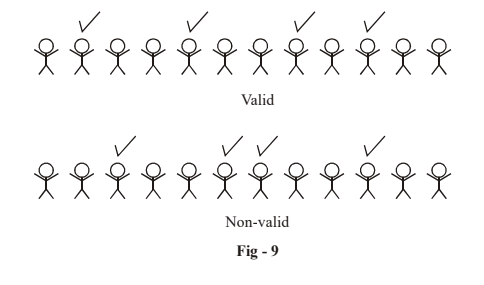We have to find some (mathematical) way of specifying a valid-selection. An obvious method that strikes is this: In our row, we represent every unselected person by U and every selected person by S. Thus, the valid selection in the figure above becomes:

$\mathbf{U\; S\; U \;U\; S\; UU \;S\; U\; S\; UU}$

To count the numbers of valid selections, we count the number of permutation of this string above, consisting of 8 “U” symbols and 4 “S” symbols, subject to the constraint that no two “S” symbols are adjacent.

To count such permutation, we first fix the 8 “U” symbols. There will then be 9 blank spaces generated for the “S” symbols as shown below:

$\mathbf{\_\_U\_\_U\_\_U\_\_U\_\_U\_\_U\_\_U\_\_U\_\_}$

From these 9 blank spaces, any 4 spaces can be chosen and the “S” symbols can be put there and it’ll be guaranteed that no two “S” symbols will be adjacent. Thus, our task now reduces to just selecting 4 blank spaces out of the 9 available to us, which can be done simply in $$^9{C_4}$$  ways.

These are the required number of selections.

Example – 14    DIVISION INTO GROUPS

We will use a standard pack of 52 cards to illustrate the concepts involved.

(a)            (i)   In how many ways can a pack of 52 cards be divided equally into 4 sets?

(ii)  Equally among 4 players?

(b)             (i)  In how many ways can this pack be divided into 4 sets of 9, 13, 14 and 16 cards each?

(ii)  Distributed among 4 players with 9, 13, 14 and 16 cards?

(c)            (i)   In how many ways can this pack be divided into 4 sets of 10, 14, 14 and 14 cards  each?

(ii)  Distributed among 4 players with 10, 14, 14 and 14 cards?

(iii)  Distributed among 4 players with 12, 12, 14 and 14 cards?

Solution:   For this question, you must understand that the division of a set into a number of subsets is different from the division of a set into a number of subsets and then distribution of those subsets to different persons. For example, suppose you are alone by yourself and you  are  dividing your deck of cards into 4 sets. What you’ll   do is do the division of the deck and keep the 4 sets in front of you. Which set lies where in front of you doesn’t matter. Your only objective was to divide the pack into 4 sets which you did. Precisely speaking, the order of the groups doesn’t matter.

On the other hand, suppose you were playing a game of Bluff with 3 other people. Before starting the game, you divide the deck into 4 sets of 13 cards each. But now you have to give each set of 13 cards to a different person. Where a set of cards goes matters. In other words, the order of the groups matters.

As another example, suppose you had to divide a class  into 3 sub-groups. You do your job-the order of the groups doesn’t matter, you just have to divide them. But suppose you had to divide the class into 3 sub-groups and place each sub-group in a different bogey of a roller coaster. In this case  the order of the groups matters because which sub-group sits in which bogey matters.

Once again, make sure that you understand very clearly when the order of the groups matters and when it doesn’t

(a) (i)   We want to divide the pack into 4 equal sets of 13 cards each. We proceed as follows;

First select a group of 13 cards from the 52 cards; call this group A. This can be done  in  $$^{52}{C_{13}}$$ ways. Group B of another 13 cards can now be selected from the remaining 39 cards in $$^{39}{C_{13}}$$  ways. Group C of another 13 cards from the remaining 26 can now be selected in  $$^{26}{C_{13}}$$ ways. This automatically leaves group D of the last 13 cards.

Thus, the division can be carried out in

$$^{52}{C_{13}} \times {\,^{39}}{C_{13}} \times {\,^{26}}{C_{13}}$$  ways.

However, notice that the order of the groups doesn’t matter, as discussed earlier. This means that even if our order of group selection was BACD or ADBC (or for that matter any other permutation of ABCD), such a selection would essentially be the same as ABCD.

The number of permutations of ABCD is 4! = 24.

Thus, the actual number of ways of division is

\begin{align}&\frac{1}{{24}}\left( {^{52}{C_{13}} \times {\,^{39}}{C_{13}} \times {\,^{26}}{C_{13}}} \right)\\=& \frac{1}{{24}}\left( {\frac{{52!}}{{13!\,\,39!}} \times \,\frac{{39!}}{{13!\,\,26!}} \times \,\frac{{26!}}{{13!\,\,13!}}} \right)\\ =& \frac{{52!}}{{{{\left( {13!} \right)}^4} \cdot 4!}} \end{align}

(ii)  If we had to distribute the cards equally among 4 players, the order of group selection would have mattered. For example, ABCD would be different from BACD. Thus, we don’t divide by 4! in this case. The number of possible distributions is \begin{align}\frac{{52!}}{{{{\left( {13!} \right)}^4}}}\end{align}

(b) (i) For this part, observe that the group sizes are all unequal. This is a somewhat different situation than the previous part where the group sizes were equal. We’ll soon see why.

We follow a similar sequence of steps as described earlier.

(i)  Select group A of 9 cards from the deck of 52 :  $$^{52}{C_9}$$ ways

(ii)  Select group B of 13 cards from the remaining 43 :  $$^{43}{C_{13}}$$ ways

(iii) Select group C of 14 cards from the remaining 30 :  $$^{30}{C_{14}}$$ ways.

(iv) This automatically leaves group D of the remaining 16 cards.

The number of ways for this division is

\begin{align}&\;\;^{52}{C_9} \times {\,^{43}}{C_{13}} \times {\,^{30}}{C_{14}}\\ &= \frac{{52!}}{{9!\,\,42!}} \times \frac{{43!}}{{13!\,\,30!}} \times \frac{{30!}}{{14!\,\,16!}}\\&= \frac{{52!}}{{9!\,\,13!\,\,14!\,\,16!}}\left\{ \begin{array}{l}{\text{From now on,note and remember that }}\\^{m + n + p + q}{C_m} \times {\,^{n + p + q}}{C_n} \times {\,^{p + q}}{C_p} \times {\,^q}{C_q}\\ = \frac{{(m + n + p + q)}}{{m!\,\,n!\,\,p!\,\,q!}}\end{array} \right\}\end{align}

Why don’t we divide by 4! here as we did earlier, even when the order of groups doesn’t   matter? This is because the group sizes are unequal. A particular selection in a particular order, say ABCD, will never be repeated in any other order of selection.

Our order of selection (in terms of group size) is 9, 13, 14 and 16 cards. Carefully think about it; a particular selection like ABCD will be done only once (and not 4! times as in the previous part due to equal group sizes)

(ii)  If we had to distribute the 4 sets to 4 players, the situation changes because which subset of cards goes to which player matters. A particular group of sets of cards, say ABCD, can be distributed among the 4 players in 4! ways. Thus, now the number of distributions will be

\begin{array}{l}\left( \begin{array}{l} {\rm{no}}{\rm{. of \;ways \;of \; division \;of \;the }}\\{\rm{deck\; as\; specified \;into \;the \;4 \;sets}}\end{array} \right)\,\,\,\, \times \,\,\,\left( \begin{array}{l}{\rm{no}}{\rm{. of \;ways\; of\; Distribution \;}}\\{\rm{of those\; sets \;to \;the\; 4\; players}}\end{array} \right)\\= \begin{align}\left( {\frac{{52!}}{{9!\,\,\,13!\,\,\,14!\,\,\,16!}}} \right)\end{align} \times \left( {4!} \right)\end{array}

(c)  (i)   In this part, the situation is a hybrid of the previous two parts. 3 of the 4 groups are equal in size while the 4th  is different.

We first select 10 cards out of 52. This can be done in  $$^{52}{C_{10}}$$ ways. The remaining 42 cards can divided into 3 equal groups (by the logic of  part -(a)) in

$\frac{{42!}}{{{{(14!)}^3}}} \times \frac{1}{{3!}}$

(Division by 3! is required since the order of groups doesn’t  matter. Thus, a particular order of 15-card groups, say ABC, is, for example, the same as ACB)

Thus, the required number of ways is

\begin{align}&^{52}{C_{10}} \times \frac{{42!}}{{{{(14!)}^3}}} \times \frac{1}{{3!}}\\&= \frac{{52!}}{{10!{{(14!)}^3}}} \times \frac{1}{{3!}}\qquad\qquad\qquad\qquad...\left( 1 \right)\end{align}

(ii)  For the second question where we have 4 players and we want to give them 10, 14, 14 and 14 cards, we already have obtained the number of possible division in (1). To distribute the subsets, each division of 4 sets can be given to the 4 players in 4! ways. Thus,  the number of distributions of the 4 subsets is

$= \frac{{52!}}{{10!{{(14!)}^3}}} \times \frac{1}{{3!}} \times 4! = \frac{{4 \times 52!}}{{10!{{\left( {14!} \right)}^3}}}$

We can also look at this current problem in the following way. We want to distribute the 52 cards to the 4 players in sets of 10, 14, 14 and 14.

We first decide which guy to give the set of 10 cards. This can be done in 4 ways. Now we choose 10 cards for him (in possibly $$^{52}{C_{10}}$$   ways). The other three players will now get the remaining 42 cards equally. We can select 12 cards for one of them in $$^{42}{C_{14}}$$  ways. The third one can get another 14 cards in  $$^{28}{C_{14}}$$ ways and finally the fourth one gets the remaining 14 cards.

Thus, the number of possible ways of distribution is $$4 \times {\,^{52}}{C_{10}} \times {\,^{42}}{C_{14}} \times {\,^{28}}{C_{14}}$$

$= \frac{{4 \times 52!}}{{10!\,\,{{(14!)}^3}}}$

(iii)             Finally, we now have two sets of one size and two of another size. We first just carry out the division into subsets, without assigning any subset to any player.

This can be done in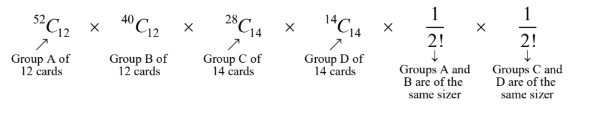\begin{align}= \frac{{52!}}{{{{(12!)}^2}{{(14!)}^2}}} \times \frac{1}{{{{\left( {2!} \right)}^2}}}\end{align}

Once the division of the deck into the 4 subsets has been accomplished, we assign a subset to each of the 4 players. This can be done in 4! ways.

Therefore, the possible number of ways of distribution of the cards to the 4 players is

$\frac{{52!}}{{{{(12!)}^2}{{(14!)}^2}}} \times \frac{1}{{{{\left( {2!} \right)}^2}}} \times 4!$

Example – 15        CIRCULAR PERMUTATIONS

In this example, we talk about circular permutations, i.e., arrangements in a circular fashion

(a)  In how many ways can n people be seated around a circular table?

(b) We have a group of 5 men and 5 women. In how many ways can we seat this group around a circular table such that:

(i) all the 5 women sit together         (ii) no two women sit together.

(c)  In how many ways can a necklace be formed from n different beads?

Solution:   Circular permutations are somewhat different from linear permutations. Lets see why.

Consider the letters A, B, C and D. The 4 linear permutations ABCD, BCDA, CDAB and DABC correspond to a single circular permutation as shown in the figure below: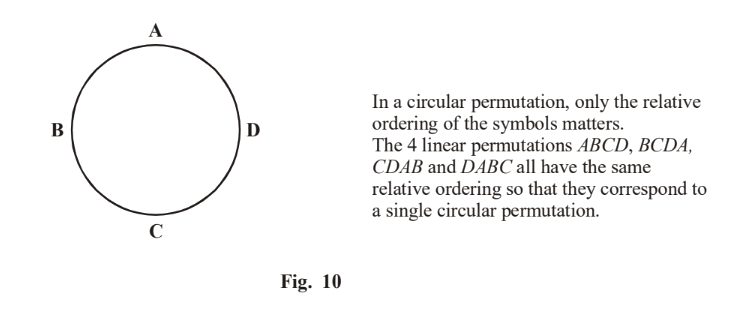This means that the number of circular permutations of ABCD is only \begin{align}\frac{{4!}}{4} = 3! = 6\end{align}

(a)  We have n people, say $${P_1},{P_2}............{P_n}.$$   As just described, n linear permutations of these people will correspond to a single circular permutation as depicted in the figure below: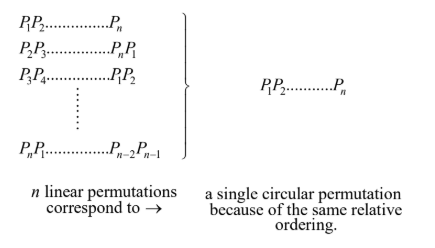Thus, the number of circular permutations is \begin{align}\frac{{n!}}{n} = (n - 1)!\end{align}

We can also arrive at this number in another way.  We take a particular person, say $${P_1}$$ , and seat him anywhere on the table. Once $${P_1}'s$$  seat becomes fixed, the rest of the (n – 1) seats bear a fixed relation to $${P_1}'s$$ . In other  words, once $${P_1}'s$$ seat becomes fixed, we can treat the (n – 1) seats left as a linear row of (n –1) seats. Thus, the remaining (n –1) people can be seated in    (n –1)! ways.

(b) Let the 5 women be represented by   $${W_1},{W_2},{W_3},{W_4},{\text{ and }}{W_5}$$  and the 5 men by $${M_1},{M_2},{M_3},{M_4},{\text{ and }}{M_5}$$ . Since we need all the women to sit together, we first treat all the 5 women as a single entity  W. Now, the 5 men and the entity W can be seated around the table in  $$\left( {(5 + 1) - 1} \right)!$$ ways, i.e., 120 ways.

(i)   Once the 5 men and the entity W have been seated, we now permute the 5 women inside the entity W. This can be done in 5! = 120 ways. The total number of ways is thus   120 × 120 = 14400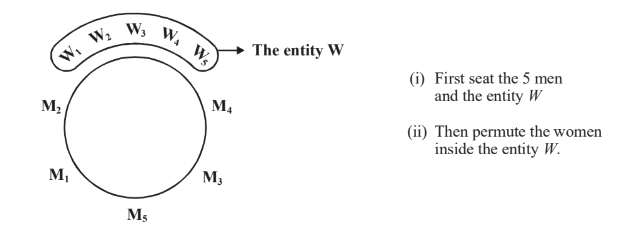Fig. 11

(ii)  Since we want no two women to sit together, we first seat all the 5 men, which can be done in (5 –1)! = 24 ways. Seating the 5 men first creates 5 non-adjacent seats where the women can then be seated in 5! = 120 ways.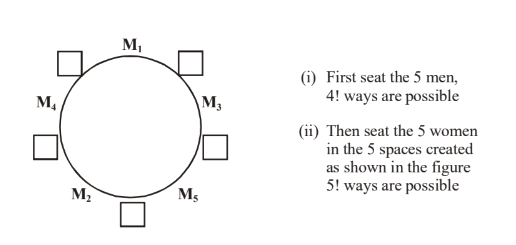Fig. 12

The total number of ways is this 5! × 4!.

(c)  The situation of a necklace is slightly different than that of seating people around a circular table. The reason is as follows:

Suppose we have 5 beads A, B, C, D and E. Consider two circular necklaces of these 5 beads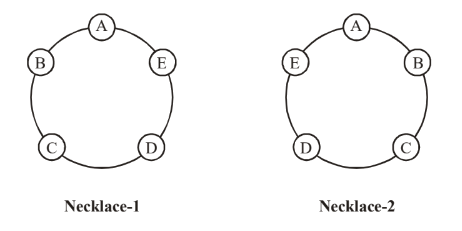Fig. 13

Are  these two necklaces different? No, because a-necklace can be worn from both ways. Necklace-2 is the same as necklace-1 if I look into it from the other side of the page. In other words, for a necklace, a clockwise permutation and its corresponding anti-clockwise permutation are identical. Thus, the number of circular permutations would reduce by a factor of two, i.e., the number of different necklaces possible is \begin{align}\frac{1}{2}(n - 1)!\end{align}.

## TRY YOURSELF - I

Q. 1 How many words can be made with the letters of the word “TECHNOLOGY” which do not begin  with T but end with Y ?

Q. 2 In how many ways can the letters of the word CINEMA be arranged so that the order of vowels do not change?

Q. 3 How many sides are there in a polygon which has 35 diagonals?

Q. 4 In how many ways can 6 boys and 4 girls sit in a row so that no boy is between two girls?

Q. 5 How many words can be made with the letters of the word BHARATI so that all the vowels are consecutive?

Q. 6 Let $$n \in N$$  and 300 < n < 3000. If n  is made of distinct digits by taking from 0, 1, 2, 3, 4, 5 then find the greatest possible number of values of n.

Q. 7 How many words can be made with the letters of the word INSTITUTION such that vowels and consonants alternate?

Q. 8 How many different committees of 5 members can be formed from 6 men and 4 ladies if each committee is to contain at least one lady?

Q. 9 Six Xs are to be placed in the squares of the given figure, such that each row contains at least one X. In how many different ways can this be done?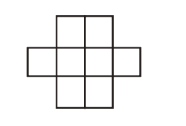Q. 10 In how many ways can 16 identical mangoes be distributed among 4 persons if none gets less than 3 mangoes?

Learn from the best math teachers and top your exams

• Live one on one classroom and doubt clearing
• Practice worksheets in and after class for conceptual clarity
• Personalized curriculum to keep up with school